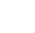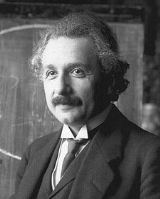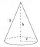xAlbert Einstein
Length Contraction MMX (10/16/2010)
Posts  1 - 2  of  2butch11735
By Thorntone E. Murray
Houston, TX

Problem

For two identical light clocks in the same reference frame to be observed to have unequal lengths is contradictory to the constancy of light speed.

Hypothesis

Identical light clocks within a reference frame are always observed to have equal length as observed from any reference frame. Constancy of the speed of light and the formula VT=D – velocity multiplied by time equals distance – are the primary basis of this hypothesis.

Thought Experiment

There is a sphere within reference frame K’. Within the sphere there are two identical light paths that are perpendicular to each other. One end of each light path is the center point of the sphere. The other end of each light path is its point of intersection with the surface of the sphere. The length of the light paths and the radius of the sphere are equal. The equal light paths are the equivalent of identical single cycle light clocks. The sphere as well as the light paths are absolutely stationary with respect to reference frame K’. Reference frame K’ is in motion at an arbitrary constant velocity relative to reference frame K which is > than 0 and < C (light speed). The relative velocity of K’ has no affect on the appearance of the sphere and light paths as observed from within K’. The x-axis of K’ is parallel to the direction of motion relative to K. One of the light paths, D’x, is parallel to and the other light path, D’y, is perpendicular to the x-axis of K’.

Within K’, the center point of the sphere produces a light pulse which radiates into the equal light paths. The end points of the respective light paths at the surface of the sphere reflect the light pulses back to the center point. The light pulses arrive at the center point of the sphere concurrently and cause the center point to illuminate distinctively. The time interval for the light pulses to traverse the equal light paths is equal (T’x = T’y). The distinctive illumination is observable from within reference frame K’ as well as from any reference frame from which the sphere is observable. A distinctive illumination of the center point occurs if and only if both reflected light pulses reach the center point concurrently, that is if and only if T’x = T’y.

Within the sphere within K’ it is given that D’x = D’y and T’x = T’y. C is constant.

Without exception: Emission of a light pulse from the center point of the sphere results in a distinctive illumination of the center point, verification that T’x = T’y.
Without exception: The distinctive illumination is observable from within K’.
Without exception: The distinctive illumination is observable from any reference frame from which the sphere is observable independent of the relative velocity of the reference frames.

Data

(D) D’x = light path length parallel to the direction of motion.
(D) D’y = light path length perpendicular to the direction of motion.
(T) T’x = time interval for the D’x light pulse to radiate from and reflect back to center point
(T) T’y = time interval for the D’y light pulse to radiate from and reflect back to center point
(V) = C = the constant speed of light

Reference frame K’ is in motion at a constant velocity > 0 but < C relative to “at rest” reference frame K. The particular relative velocity is of no consequence. A light pulse is emitted from the center point of the sphere within K’. The distinctive illumination is necessarily observed from within K’ verifying the reflected pulses arrived at the center point of the sphere concurrently. The distinctive illumination is also observed from reference frame K. Therefore as observed from “at rest” reference frame K T’x = T’y. Of course, C is constant. Time delay and/or Doppler shift have no bearing in the matter.

As observed from “at rest” reference frame K:
(V) Velocity multiplied by (T) Time equals (D) Distance or VT=D
C * T’x = D’x
C * T’y = D’y
substitute T’x for T’y
C * T’x = D’x
C * T’x = D’y
Therefore D’x = D’y

T’ is equal for both as verified by a distinctive illumination. C is equal for both. It is therefore unequivocal that D’ is the same for both as observed from the “rest” frame.

As observed from reference frame K at relative rest, D’y, the length of the light path perpendicular to the direction of motion and D’x, the length of the light path parallel to the direction of motion are equal. Therefore, length parallel to the direction of motion did not contract relative to length perpendicular to the direction of motion. This finding directly contradicts Lorentz contraction.

The famous Michelson - Morley experiment and other experiments of the kind were considered to have failed with null results because the fringe shifts detected failed to approach the expected magnitudes. More importantly, for these intents and purposes, the lengths of the arms of the various interferometers had no detectable variations in length with respect to each other. Those results are consistent with and support the finding herein disclosed.

Conclusion

Equal lengths within a reference frame are always equal as observed from any reference frame independent of the relative velocity and/or orientation to the direction of motion.

Prediction

An actual experiment based on the one described herein will measure the rotational velocity and angle of the rotation of the earth relative to its stationary gravitational reference frame. Also, Lorentz transformation will be conclusively disproved.
replied to:  butch11735TrevorWhite
Replied to:  By Thorntone E. Murray Houston, TX Problem For...
Just a small point here these/this effect is only significant at speeds approaching the speed of light, measuring it within the bounds of earth even using light as your arms does not mean you are measuring it at these speeds unless you are suggesting accelerating your experiment, the whole thng, to near light speed.